# For the given stale of stress, determine (a) the orientation of the planes of maximum in-plane shearing stress, (b) the maximum in-plane shearing stress, (c) the corresponding normal stress.

Question-AnswerCategory: Strength of MaterialsFor the given stale of stress, determine (a) the orientation of the planes of maximum in-plane shearing stress, (b) the maximum in-plane shearing stress, (c) the corresponding normal stress.

For the given stale of stress, determine (a) the orientation of the planes of maximum in-plane shearing stress, (b) the maximum in-plane shearing stress, (c) the corresponding normal stress.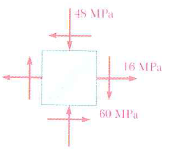Step: 1

(a)
Calculate the orientation of the planes of the maximum in-plane shearing stress using the following formula:Here, normal stress in the x-direction is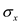, normal stress in the y-direction is, and the shear stress is.
Substitute,, andin the above equation.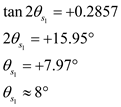Calculate the second shear plane angle by using the following relation:Therefore, the orientation of the planes of the maximum in-plane shearing stress are.

Step: 2

(b)
Calculate the maximum in plane shearing stress using the following formula:Therefore, the maximum in-plane shearing stress is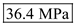.

Step: 3

(c)
Calculate the normal stress on the maximum shear stress plane:
Note: the normal stress on the maximum shear stress plane is equal to the average of the two normal stresses.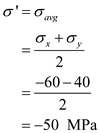Therefore, the normal stress on the maximum shear stress plane is.